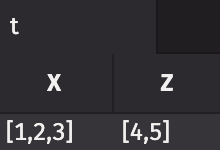Version: Python

# How to group and ungroup data

This guide will show you how to group and ungroup table data in Deephaven.

This guide uses a table of apple data called `apples` created using `new_table`. Many of the grouping and ungrouping examples use this table. If you are unfamiliar with creating tables from scratch using `new_table`, please see our guide Create a new table.

Use the code below to create the `apples` table:

``from deephaven import new_tablefrom deephaven.column import string_col, int_colapples = new_table([    string_col("Type", ["Granny Smith", "Granny Smith", "Gala", "Gala", "Golden Delicious", "Golden Delicious"]),    string_col("Color", ["Green", "Green", "Red-Green", "Orange-Green", "Yellow", "Yellow"]),    int_col("WeightGrams", [102, 85, 79, 92, 78, 99]),    int_col("Calories", [53, 48, 51, 61, 46, 57])])``

## Group data with `group_by`​

The `group_by` method groups columnar data into arrays. A list of grouping column names defines grouping keys. All rows from the input table with the same key values are grouped together.

If no input is supplied to `group_by`, then there will be one group, which contains all of the data. The resultant table will contain a single row, where column data is grouped into a single array. This is shown in the example below:

``applesByNoColumn = apples.group_by()``

If a single input is supplied to `group_by`, then the resultant table will have row data grouped into arrays based on each unique value in the input column. This is shown in the example below:

``applesByType = apples.group_by(by=["Type"])``

If more than one input is supplied to `group_by`, then the resultant table will have row data grouped into arrays based on unique value pairs from the grouping columns. This is shown in the example below:

``applesByTypeAndColor = apples.group_by(by=["Type", "Color"])``

Formulas supplied to `group_by` can also be used to both add new columns and group data. The example below simultaneously adds two new columns and groups on the columns. If you are unfamiliar with the ternary conditional statements used in the example, see How to use the ternary conditional operator.

``applesByClassAndDiet = apples.group_by(by=["Class = (WeightGrams < 90) ? `Light` : `Heavy`",    "Diet = (Calories < 50) ? `Allowed` : `Not Allowed`"])``

## Ungroup data with `ungroup`​

The `ungroup` method is the inverse of `group_by`. It unwraps content from arrays and builds a new set of rows from it. The method takes optional columns as input. If no inputs are supplied, all array columns are unwrapped. If one or more columns are given as input, only those columns will have their array values unwrapped into new rows.

The example below shows how `ungroup` reverses the `group_by` operation used to create `applesByClassAndDiet` when no columns are given as input. Notice how all array columns have been unwrapped, leaving a single element in each row of the resultant table:

``newApples = applesByClassAndDiet.ungroup()``

The example below uses `ungroup` to unwrap the `Color` column in `applesByClassAndDiet`. This unwraps only arrays in the `Color` column, and not the others. Notice how the `Type`, `WeightGrams`, and `Calories` columns still contain arrays:

``applesUngroupedByColor = applesByClassAndDiet.ungroup(["Color"])``

## Different array types​

The `ungroup` method can ungroup DbArrays and Java arrays.

The example below uses the `empty_table` method to create a table with two columns and one row. Each column contains a Java array with 3 elements. The `ungroup` method works as expected on Java arrays.

``from deephaven import empty_tablet = empty_table(1).update(formulas=["X = new int[]{1, 2, 3}", "Z = new int[]{4, 5, 6}"])t_ungrouped = t.ungroup()``
``from deephaven import new_tablefrom deephaven.column import int_colt = new_table([    int_col("X", [1, 2, 3])]).group_by().update(formulas=["Z = new int[]{4, 5, 6}"])t_ungrouped = t.ungroup()``

## Different array lengths​

The `ungroup` method cannot unpack a row that contains arrays of different length.

The example below uses the `empty_table` method to create a table with two columns and one row. Each column contains a Java array, but one has three elements and the other has two. Calling `ungroup` without an input column will result in an error.

``from deephaven import empty_tablet = empty_table(1).update(formulas=["X = new int[]{1, 2, 3}", "Z = new int[]{4, 5}"])t_ungrouped = t.ungroup() # This results in an error``It is only possible to ungroup columns of the same length. Arrays of different lengths must be ungrouped separately.

``from deephaven import empty_tablet = empty_table(1).update(formulas=["X = new int[]{1, 2, 3}", "Z = new int[]{4, 5}"])t_ungroupedByX = t.ungroup(["X"])t_ungroupedByZ = t.ungroup(["Z"])``

## Null values​

Using `group_by` on a table with null values will work properly. Null values will appear as empty array elements when grouped with `group_by`. Null array elements unwrapped using `ungroup` will appear as null (empty) row entries in the corresponding column.

The example below uses the `empty_table` method and the ternary operator to create a table with two columns of 5 rows. The first and second rows contain null values. Null values behave as expected during grouping and ungrouping.

``from deephaven import empty_tablefrom deephaven.constants import NULL_INTt = empty_table(5).update(formulas=["X = i", "Z = i < 2 ? NULL_INT : i-2"])t_by = t.group_by()new_t = t_by.ungroup()``

The example below uses the `empty_table` method to create a table with one column and one row. The single cell in the table contains a null Java array. Calling `ungroup` on this table results in an empty table with one column.

``from deephaven import empty_tablet = empty_table(1).update(formulas=["X = (int[])(null)"])t_ungrouped = t.ungroup()``